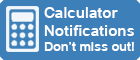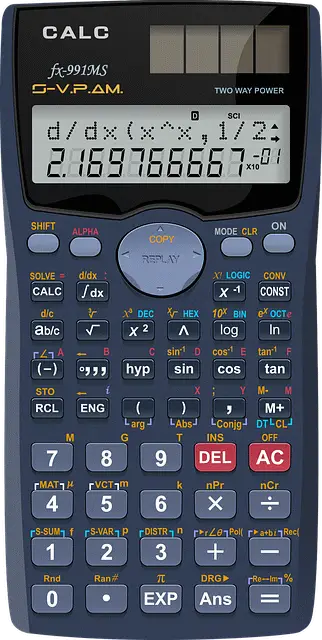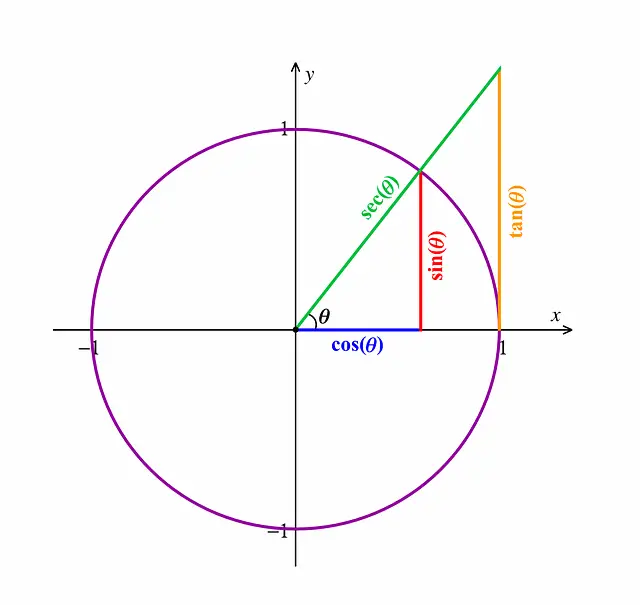Calculator Library
Free Online CalculatorsHome » 87 Calculators » Blog » How To Find CSC On A Calculator

Huge Domain Name Sale | Free Domains
Domain names on sale at crazy prices. Register a new domain for FREE when you buy another service.

## HOW TO FIND CSC ON A CALCULATORFinding the cosecant function on a calculator can be tricky, but with a little practice, it can be easy to do. In this article, we'll show you how to find the cosecant function on your calculator and how to use it in different equations. We'll also provide some helpful tips that will make using the cosecant function easier for you!

## What Is A Scientific Calculator?

A scientific calculator is a type of calculator designed to perform mathematical operations that are more complicated than those that can be performed on a standard calculator. Scientific calculators usually have more buttons and features than standard calculators and they often look different too.

The cosecant function is one of the many functions that you can find on a scientific calculator. Other functions that you might find on a scientific calculator include the sine, cosine and tangent functions.

## What Is CSC Function?

The cosecant function is the reciprocal of the sine function. This means that the cosecant of an angle is equal to the ratio of the length of the hypotenuse to the length of the opposite side. The cosecant function is often abbreviated as "CSC."

## How To Use The CSC Function?Now that you know how to find the cosecant function on your calculator, let's take a look at how to use it in different equations. The cosecant function is also very useful in trigonometry. Trigonometry is the branch of mathematics that deals with the relationships between angles and sides of triangles.

The cosecant function can be used to solve for angles and sides in right triangles. To use the cosecant function, you'll need to know the length of one side of the triangle and the length of the hypotenuse. Once you have these two values, you can plug them into the equation or cosecant formula below:

cosecant (angle) = hypotenuse / opposite side

For example, let's say that you're trying to find the cosecant of an angle in a right triangle. You know that the length of the hypotenuse is 15 and the length of the opposite side is 12. Plugging these CSC values into the equation or CSC formula above, we get:

cosecant (angle) = 15 / 12

This means that the cosecant of the angle is equal to 15/12. Thus we get the CSC value.

## How To Find CSC On Calculator?

There are a few different ways that you can find the cosecant function on your calculator. One way is to look for the "sin" button and then press the inverse button. This will change the sin function to the cosecant function. Another way is to first press the shift button and then press the "sin" button. This will also change the sin function to the cosecant function.

On digital or mobile calculators, you can usually find the cosecant function by looking for the "sin" button and then pressing the inverse button. This will change the sin function to the cosecant function. Some calculators directly have a cosecant button which you can press to use the function.

If you're having trouble finding the cosecant function on your calculator, you can always consult the manual that came with your calculator. This should have all of the information that you need to find and use the cosecant function.

## What Other Trigonometric Functions Can I Use?

In addition to the cosecant function, there are a few other trigonometric functions that you might find useful. These include the sine, cosine and tangent functions. Each of these functions can be used to solve for different values in a right triangle.

These are just a few of the many functions that you can find on a scientific calculator. If you're interested in learning more about calculating trigonometric functions, there are several resources available online and in print. You can also find several video tutorials that can help you learn how to use these functions.

## Popular Types Of Scientific Calculators With CSC Function

There are a few different types of cosecant calculator that you might find useful. These include the graphing calculator, the scientific calculator and the statistical calculator. Each of these calculators has its own set of features and functions.

The graphing calculator is one of the most popular types of scientific calculator. It's typically used by students in high school and college. The graphing calculator can be used to graph equations and functions. It can also be used to solve for different values.

The scientific calculator is another popular type of calculator. It's typically used by students in middle school and high school. The scientific calculator has several different features that make it useful for solving math problems. It can also be used to convert between different units of measurement.

The statistical calculator is a type of scientific calculator that's typically used by students in statistics class. The statistical calculator can be used to calculate the mean, median, mode and standard deviation. It can also be used to calculate probability.

What type of scientific calculator you choose to use is up to you.

We have two types of online CSC calculator available at Calculatorlibrary.com:

- Advanced Scientific Calculator: This calculator can be used like a normal handheld scientific calculator. It can solve equations, basic arithmetic operations, trigonometric functions and more.

- iPhone Scientific Calculator: This calculator is based on the widely-used iPhone(iOS) platform. It has a sleek and modern design. The calculator can solve equations, basic arithmetic operations, trigonometric functions and more.

You can also find a variety of scientific calculators with CSC functions available for purchase online and in stores.

## Conclusion

A cosecant function is a useful tool that you can use to solve math problems. You can find the cosecant function on most scientific calculators. If you're having trouble finding the cosecant function, you can consult the manual that came with your calculator. You can also use the inverse button to change the sin function to the cosecant function. There are several different types of scientific calculators that you might find useful. These include the graphing calculator, the scientific calculator and the statistical calculator.

 Previous Post« How To Do Fractions On A Calculator? Next PostHow To Plot Points On A Graphing Calculator? »